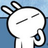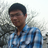首页   注册   登录
V2EX = way to explore
V2EX 是一个关于分享和探索的地方

V2EX  ›  数学

# 如何理解递归，如何清晰地利用递归在不背算法模板的条件下写出程序？

DIJ · 2015-07-19 17:08:32 +08:00 · 2535 次点击

```int quickpower(int a,int x)
{
if (x==0) return 1;
int r=quickpower(a,x/2);
r=r*r;
cout <<x<<endl;
if(x%2!=0) r*=a;
return r;
}
```

r=quickpower(3,1)
quickpower(3,1)=quickpower(3,0)*quickpower(3,0)=1
......
r=1

```void full_permutation(int depth)
{
if(depth==n+1)
{
for(int i=1;i<=n;i++)
cout <<item[i]<<" ";
cout <<endl<<endl;
return ;
}
for(int j=1;j<=n;j++)
if(used[j]==0)
{
used[j]=1;
item[depth]=j;
//cout <<"depth="<<depth<<endl;
//cout <<"used["<<j<<"]="<<used[j]<<endl;
full_permutation(depth+1);
used[j]=0;
}
}
```

depth=1
used=1
depth=2
used=1
depth=3
used=1
1 2 3

depth=2
used=1
depth=3
used=1
1 3 2

depth=1
used=1
depth=2
used=1
depth=3
used=1
2 1 3

......
321

5 回复  |  直到 2015-07-24 09:29:22 +08:001 sandideas   2015-07-19 18:03:37 +08:00 没时间看。。只能说快速幂的原理应该是这样的。假如要求3的10次方，等于3的5次方*3的5次方。3^5=3^2*3^2*3^1.。。递归就是这么走的。2 sandideas   2015-07-19 18:11:22 +08:00 via iPhone 顺便说一下。。不会算到3^0次方。。因为最后有个判断3 jiang42   2015-07-19 18:42:01 +08:00 递归的优点之一就是写出的程序清晰啊。。。4 dorentus   2015-07-19 19:35:31 +08:00 via iPhone if(x%2!=0) r*=a 被你漏掉了5 vwok   2015-07-24 09:29:22 +08:00 if (x==0) return 1; int r=quickpower(a,x/2)*quickpower(a,x-x/2);按照实际算法思路来就好了

World is powered by solitude
VERSION: 3.9.8.3 · 19ms · UTC 23:24 · PVG 07:24 · LAX 16:24 · JFK 19:24
♥ Do have faith in what you're doing.
 沪ICP备16043287号-1